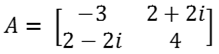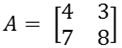MORE IN Applied Mathematics 4
MU Computer Engineering (Semester 4)
Applied Mathematics 4
May 2013
Total marks: --
Total time: --
INSTRUCTIONS
(1) Assume appropriate data and state your reasons
(2) Marks are given to the right of every question
(3) Draw neat diagrams wherever necessary

1 (a) Check if the following function is harmonic.
f(γ,θ) = [γ + (a2/γ)] cosθ
5 M
1 (b) Integrate function f(z) = x2 + iy from A (1,1) to B (2,4) along the curve x=t, y=t2
5 M
1 (c) Prove that the eigen values of an orthogonal matrix are +1 or -1.
5 M
1 (d) Construct the dual of the following LPP.
Maximise z = x1 + 3x2 - 2x3 + 5x4;
subject to 3x1 - x2 + x3 -4x4 = 6;
5x1 + 3x2 - x3 - 2x4 = 4;
x1, x3 ≥ 0; x3, x4 unrestricted.
5 M

2 (a) Evaluate:where C is a circle |z|=1.
6 M
2 (b) Diagonalise the hermitian matrix6 M
2 (c) Use simplex method to solve the LPP
Maximize z = 1000x1 + 4000x2 + 5000x3 subject to the constraints:
x1 + 2x2 + 3x3≤ 14
x1 + 2x1 ≤ 14
x1, x2, x3 ≥ 0
8 M

3 (a) Evaluate using contour integration:6 M
3 (b) State caley-hamilton theorem. Use it to find A-1 and A4
where,6 M
3 (c) Use penalty method to
Minimize z = x1 + 2x2 + x3
subject to x1 + (x2/2) + (x3/2) ≤ 1
(3/2) x1 + 2x2 + x3 ≥ 8
x1, x2,x3 ≥ 0
8 M

4 (a) Find A100
where,6 M
4 (b) If f(z) is analytic function, prove that6 M
4 (c) Use dual simplex method to solve the LPP.
Minimize z = 3x1 + 2x2 + x3 + 4x4;
subject to 2x1 + 4x2 + 5x3 + x4 ≥ 10
3x1 - x2 + 7x3 - 2x4 ≥ 2
5x1 + 2x2 + x3 + 6x4 ≥ 15
x1, x2, x3, x4 ≥ 0
8 M

5 (a) Find the bilinear transformation that maps the points 1, -1, 2 in z-plane onto the points 0, 2, -i in w-plane.
6 M
5 (b) Is A derogatory?6 M
5 (c) Evaluate:where 0 < b < a.
8 M

6 (a) If A = where a, b, c are positive integers, then prove that
(i) a + b + c is an eigen value of A and
(ii) if A is non-singular, one of the eigen values is negative.
6 M
6 (b) Find the image of region bounded by x=1, y=1, x+y=1 under the transformation w=z2.
6 M
6 (c) Use Lagrangian Multiplier method to optimize (8)z=2x12 + x22 + 3x32 + 10x1 + 8x2 + 6x3 - 100;
subject to x1 + x2 + x3 = 20
x1, x2, x3 ≥ 0
8 M

7 (a) Obtain two Laurent s series for 1/[(z-1)(z-2)] in the regions:(i) 1 < |z-1|< 2
(ii) 1 < |z-3|< 2
6 M
7 (b) Find the analytic function f(z) whose imaginary part is
e-x {2xy cos y + (y2 - x2) sin y}.
6 M
7 (c) Solve the following N.L.P.P. using Kuhn-Tucker conditions.
Optimize z = 2x1 + 3x2 - (x12 + x22 + x32)
subject to x1 + x2 ≤ 1
2x1 + 3x2 ≤ 6
x1 , x2 ≥ 0.
8 M

More question papers from Applied Mathematics 4